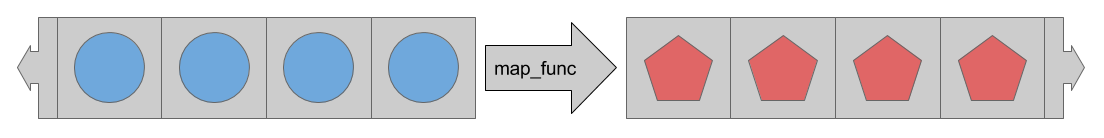# 数据集：快速了解

`tf.data`

• 从 numpy 数组读取内存数据。
• 逐行读取 csv 文件。

## 基本输入

``````def train_input_fn(features, labels, batch_size):
"""一个用来训练的输入函数"""
# 将输入值转化为数据集。
dataset = tf.data.Dataset.from_tensor_slices((dict(features), labels))

# 混排、重复、批处理样本。
dataset = dataset.shuffle(1000).repeat().batch(batch_size)

# 返回数据集
return dataset
``````

### 参数

• `features`：一个形如 `{'feature_name':array}` 的数据字典（或者是 `DataFrame`），它包含了原始的输入特征。
• `labels`：一个包含每个样本的 label 的数组。
• `batch_size`：一个指示所需批量大小的整数。

`premade_estimator.py` 中，我们使用 `iris_data.load_data()` 函数来检索虹膜数据。

``````import iris_data

# 获取数据
features, labels = train
``````

``````batch_size=100
iris_data.train_input_fn(features, labels, batch_size)
``````

### （数组）片段

TF Layers 教程：构建卷积神经网络

``````train, test = tf.keras.datasets.mnist.load_data()
mnist_x, mnist_y = train

mnist_ds = tf.data.Dataset.from_tensor_slices(mnist_x)
print(mnist_ds)
``````

``````<TensorSliceDataset shapes: (28,28), types: tf.uint8>
``````

``````dataset = tf.data.Dataset.from_tensor_slices(dict(features))
print(dataset)
``````
``````<TensorSliceDataset

shapes: {
SepalLength: (), PetalWidth: (),
PetalLength: (), SepalWidth: ()},

types: {
SepalLength: tf.float64, PetalWidth: tf.float64,
PetalLength: tf.float64, SepalWidth: tf.float64}
>
``````

iris 的第一行 `train_input_fn` 使用相同的功能，但是增加了一层结构。它创建了一个包含 `(features_dict, label)` 数据对的数据集。

``````# 将输入转化为数据集。
dataset = tf.data.Dataset.from_tensor_slices((dict(features), labels))
print(dataset)
``````
``````<TensorSliceDataset
shapes: (
{
SepalLength: (), PetalWidth: (),
PetalLength: (), SepalWidth: ()},
()),

types: (
{
SepalLength: tf.float64, PetalWidth: tf.float64,
PetalLength: tf.float64, SepalWidth: tf.float64},
tf.int64)>
``````

### 操作

``````# 样本的混排、重复、批处理。
dataset = dataset.shuffle(1000).repeat().batch(batch_size)
``````

`tf.data.Dataset.shuffle`

`tf.data.Dataset.repeat`

`tf.data.Dataset.batch`

``````print(mnist_ds.batch(100))
``````
``````<BatchDataset
shapes: (?, 28, 28),
types: tf.uint8>
``````

`train_input_fn` 中，批处理之后，`数据集` 包含元素们的一维向量，这些一维向量的前面部分是：

``````print(dataset)
``````
``````<TensorSliceDataset
shapes: (
{
SepalLength: (?,), PetalWidth: (?,),
PetalLength: (?,), SepalWidth: (?,)},
(?,)),

types: (
{
SepalLength: tf.float64, PetalWidth: tf.float64,
PetalLength: tf.float64, SepalWidth: tf.float64},
tf.int64)>
``````

## 读取 CSV 文件

`tf.data`

``````import iris_data
``````

`iris_data.csv_input_fn` 函数包括了一个用 `Dataset` 解析 csv 文件的替代方案。

### 建立 `Dataset`

`tf.data.Dataset.skip`

``````ds = tf.data.TextLineDataset(train_path).skip(1)
``````

### 建立一个 csv 行解析器

`tf.decode_csv`

`tf.decode_csv`

``````# 描述文本列的元数据
COLUMNS = ['SepalLength', 'SepalWidth',
'PetalLength', 'PetalWidth',
'label']
FIELD_DEFAULTS = [[0.0], [0.0], [0.0], [0.0], ]
def _parse_line(line):
# 将行解码到 fields 中
fields = tf.decode_csv(line, FIELD_DEFAULTS)

# 将结果打包成字典
features = dict(zip(COLUMNS,fields))

# 将标签从特征中分离
label = features.pop('label')

return features, label
``````

### 解析多行

`tf.data.Dataset.map``tf.data.Dataset.map`

``````ds = ds.map(_parse_line)
print(ds)
``````
``````<MapDataset
shapes: (
{SepalLength: (), PetalWidth: (), ...},
()),
types: (
{SepalLength: tf.float32, PetalWidth: tf.float32, ...},
tf.int32)>
``````

`iris_data.csv_input_fn` 函数的余下部分和 Basic input 中介绍的 `iris_data.train_input_fn` 函数相同。

### 实践

``````train_path, test_path = iris_data.maybe_download()

# 所有的输入都是数字
feature_columns = [
tf.feature_column.numeric_column(name)
for name in iris_data.CSV_COLUMN_NAMES[:-1]]

# 构建 estimator
est = tf.estimator.LinearClassifier(feature_columns,
n_classes=3)
# 训练 estimator
batch_size = 100
est.train(
steps=1000,
input_fn=lambda : iris_data.csv_input_fn(train_path, batch_size))
``````

Estimator 期望 `input_fn` 没有任何参数。要解除这个限制，我们使用 `lambda` 来捕获参数并提供预期的接口。